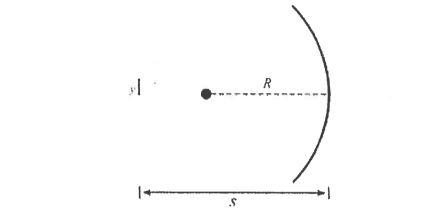# Problem: An object with height y = 2 mm is placed a distance s = 24 cm in front of the vertex of a concave spherical mirror with radius of curvature R = 16 cm, as shown in the figure below. On the figure, draw and label any two principal rays.

###### Problem Details

An object with height y = 2 mm is placed a distance s = 24 cm in front of the vertex of a concave spherical mirror with radius of curvature R = 16 cm, as shown in the figure below.

On the figure, draw and label any two principal rays.